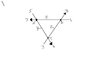# Traffic flow (Linear Algebra)

• vs55

## Homework Statement

the following statement shows the traffic flow(in cars/minute) along 3 one way streets through the intersections A, B and C
Set up and solve a system of linear equations(using the augmented matrix for the system) to help you find the flows x,y and z. Be sure to generate the RREF of the system
http://img18.imageshack.us/img18/2276/trafficflow.jpg [Broken]

## Homework Equations

traffic flow in must equal flow out

## The Attempt at a Solution

so to start things off, equations i put:
3 + z = 3
6 + x = 7
5 + y = 4
is this at all right?

#### Attachments

•trafficflow.jpg
7.9 KB · Views: 699
Last edited by a moderator:

## Homework Statement

the following statement shows the traffic flow along 3 one way streets through the intersections A, B and C
Set up and solve a system of linear equations(using the augmented matrix for the system) to help you find the flows x,y and z. Be sure to generate the RREF of the system
http://img216.imagevenue.com/img.php?image=27718_trafficflow_122_18lo.jpg

## Homework Equations

traffic flow in must equal flow out

## The Attempt at a Solution

so to start things off, equations i put:
3 + z = 3
6 + x = 7
5 + y = 4
is this at all right?

I don't think so. For your first equation, 3 + z = 3 ==> z = 0, which doesn't make any sense. How about giving us the problem exactly as it was worded? Useful information would be what the numbers in your drawing represent.

I believe that what you have as "relevant equations" means that the traffic flow into and out of an intersection must be equal.

this is the problem exactly as it was worded, but if u insist...i added "cars/minute" :|
i also added the label on the intersections in the image A,B and C
and sorry i thought the numbers were obvious :S, they represent the flow out that that arrow

i'm guessing i have to go take away some of the flow as well? I'm just not sure how to start the problem

If my previous interpretation of what traffic flow in equals traffic flow out is on track, here's what you have:
Node A (upper left intersection) x + 5 = y + 7
Node B (upper right int.) z + 6 = x + 3
Node C (lower int.) y + 3 = z + 4

Solve this system.

i got:
-x + z =-3
-z +y = 1
-y + x = z
to help set up the matrix:
-x 0 z
0 y -z
x -y 0
augmented matrix:
-1 0 1 |-3 ---->(1)
0 1 -1 |1------>(2)
1 -1 0 |2------>(3)

after some math:
(1)/-1
(3)-(1)
(3)+(2)

1 0 -1 | 3
0 1 -1 | 1
0 0 0 | 0

1x -1z = 3
1y -1z =1
z=free

z=1,y=2,x=4
is this right?

i got:
-x + z =-3
-z +y = 1
-y + x = z
The last equation should be -y + x = 2, but otherwise I got the same solution that you showed.

thx for ur help!